# NCERT Solutions for Class 9th: Ch 8 Quadrilaterals Maths

#### NCERT Solutions for Class 9th: Ch 8 Quadrilaterals Maths

Page No: 146

Exercise 8.1

1. The angles of quadrilateral are in the ratio 3 : 5 : 9 : 13. Find all the angles of the quadrilateral.

Let x be the common ratio between the angles.
Sum of the interior angles of the quadrilateral = 360°
Now,
3x + 5x + 9x + 13x = 360°
⇒ 30x = 360°
⇒ x = 12°
3x = 3×12° = 36°
5x = 5×12° = 60°
9x = 9×12° = 108°
13x = 13×12° = 156°

2. If the diagonals of a parallelogram are equal, then show that it is a rectangle.Given,
AC = BD
To show,
To show ABCD is a rectangle we have to prove that one of its interior angle is right angled.
Proof,
BC = BA (Common)
AC = AD (Opposite sides of a parallelogram are equal)
AC = BD (Given)
Therefore, ΔABC ≅ ΔBAD by SSS congruence condition.
∠A = ∠B (by CPCT)
also,
∠A + ∠B = 180° (Sum of the angles on the same side of the transversal)
⇒ 2∠A = 180°
⇒ ∠A = 90° = ∠B
Thus ABCD is a rectangle.

3. Show that if the diagonals of a quadrilateral bisect each other at right angles, then it is a rhombus.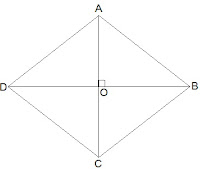Let ABCD be a quadrilateral whose diagonals bisect each other at right angles.
Given,
OA = OC, OB = OD and ∠AOB = ∠BOC = ∠OCD = ∠ODA = 90°
To show,
ABCD is parallelogram and AB = BC = CD = AD
Proof,
In ΔAOB and ΔCOB,
OA = OC (Given)
∠AOB = ∠COB (Opposite sides of a parallelogram are equal)
OB = OB (Common)
Therefore, ΔAOB ≅ ΔCOB by SAS congruence condition.
Thus, AB = BC (by CPCT)
Similarly we can prove,
AB = BC = CD = AD
Opposites sides of a quadrilateral are equal hence ABCD is a parallelogram.
Thus, ABCD is rhombus as it is a parallelogram whose diagonals intersect at right angle.

4. Show that the diagonals of a square are equal and bisect each other at right angles.Let ABCD be a square and its diagonals AC and BD intersect each other at O.
To show,
AC = BD, AO = OC and ∠AOB = 90°
Proof,
BC = BA (Common)
Therefore, ΔABC ≅ ΔBAD by SAS congruence condition.
Thus, AC = BD by CPCT. Therefore, diagonals are equal.
Now,
In ΔAOB and ΔCOD,
∠BAO = ∠DCO (Alternate interior angles)
∠AOB = ∠COD (Vertically opposite)
AB = CD (Given)
Therefore, ΔAOB ≅ ΔCOD by AAS congruence condition.
Thus, AO = CO by CPCT. (Diagonal bisect each other.)
Now,
In ΔAOB and ΔCOB,
OB = OB (Given)
AO = CO (diagonals are bisected)
AB = CB (Sides of the square)
Therefore, ΔAOB ≅ ΔCOB by SSS congruence condition.
also, ∠AOB = ∠COB
∠AOB + ∠COB = 180° (Linear pair)
Thus, ∠AOB = ∠COB = 90° (Diagonals bisect each other at right angles)

5. Show that if the diagonals of a quadrilateral are equal and bisect each other at right angles, then it is a square.Given,
Let ABCD be a quadrilateral in which diagonals AC and BD bisect each other at right angle at O.
To prove,
Proof,
In ΔAOB and ΔCOD,
AO = CO (Diagonals bisect each other)
∠AOB = ∠COD (Vertically opposite)
OB = OD (Diagonals bisect each other)
Therefore, ΔAOB ≅ ΔCOD by SAS congruence condition.
Thus, AB = CD by CPCT. --- (i)
also,
∠OAB = ∠OCD (Alternate interior angles)
⇒ AB || CD
Now,
In ΔAOD and ΔCOD,
AO = CO (Diagonals bisect each other)
∠AOD = ∠COD (Vertically opposite)
OD = OD (Common)
Therefore, ΔAOD ≅ ΔCOD by SAS congruence condition.
Thus, AD = CD by CPCT. --- (ii)
also,
⇒ AD = BC = CD = AB --- (ii)
also,  ∠ADC = ∠BCD  by CPCT.
and ∠ADC + ∠BCD = 180° (co-interior angles)
⇒ ∠ADC = 90° --- (iii)
One of the interior ang is right angle.
Thus, from (i), (ii) and (iii) given quadrilateral ABCD is a square.

6. Diagonal AC of a parallelogram ABCD bisects ∠A (see Fig. 8.19). Show that
(i) it bisects ∠C also,
(ii) ABCD is a rhombus.(i)
AD = CB (Opposite sides of a ||gm)
DC = BA (Opposite sides of a ||gm)
AC = CA (Common)
Therefore, ΔADC ≅ ΔCBA by SSS congruence condition.
Thus,
∠ACD = ∠CAB by CPCT
⇒ ∠ACD = ∠BCA
Thus, AC bisects ∠C also.

⇒ AD = CD (Opposite sides of equal angles of a triangle are equal)
Also, AB = BC = CD = DA (Opposite sides of a ||gm)
Thus, ABCD is a rhombus.

7. ABCD is a rhombus. Show that diagonal AC bisects ∠A as well as ∠C and diagonal BD bisects ∠B as well as ∠D.Let ABCD is a rhombus and AC and BD are its diagonals.
Proof,
AD = CD (Sides of a rhombus)
∠DAC = ∠DCA (Angles opposite of equal sides of a triangle are equal.)
also, AB || CD
⇒ ∠DAC = ∠BCA (Alternate interior angles)
⇒ ∠DCA = ∠BCA
Therefore, AC bisects ∠C.
Similarly, we can prove that diagonal AC bisects ∠A.

Also, by preceding above method we can prove that diagonal BD bisects ∠B as well as ∠D.

8. ABCD is a rectangle in which diagonal AC bisects ∠A as well as ∠C. Show that:
(i) ABCD is a square
(ii) diagonal BD bisects ∠B as well as ∠D.(i)∠DAC = ∠DCA (AC bisects ∠A as well as ∠C)
⇒ AD = CD (Sides opposite to equal angles of a triangle are equal)
also, CD = AB (Opposite sides of a rectangle)
Therefore, AB = BC = CD = AD
Thus, ABCD is a square.

(ii) In ΔBCD,
BC = CD
⇒ ∠CDB = ∠CBD (Angles opposite to equal sides are equal)
also, ∠CDB = ∠ABD (Alternate interior angles)
⇒ ∠CBD = ∠ABD
Thus, BD bisects ∠B
Now,
Thus, BD bisects ∠D

Page No: 147

9. In parallelogram ABCD, two points P and Q are taken on diagonal BD such that DP = BQ (see Fig. 8.20). Show that:
(i) ΔAPD ≅ ΔCQB
(ii) AP = CQ
(iii) ΔAQB ≅ ΔCPD
(iv) AQ = CP
(v) APCQ is a parallelogram

(i) In ΔAPD and ΔCQB,
DP = BQ (Given)
∠ADP = ∠CBQ (Alternate interior angles)
AD = BC (Opposite sides of a ||gm)
Thus, ΔAPD ≅ ΔCQB by SAS congruence condition.

(ii) AP = CQ by CPCT as ΔAPD ≅ ΔCQB.

(iii) In ΔAQB and ΔCPD,
BQ = DP (Given)
∠ABQ = ∠CDP (Alternate interior angles)
AB = BCCD (Opposite sides of a ||gm)
Thus, ΔAQB ≅ ΔCPD by SAS congruence condition.

(iv) AQ = CP by CPCT as ΔAQB ≅ ΔCPD.

(v) From (ii)  and (iv), it is clear that APCQ has equal opposite sides also it has equal opposite angles. Thus, APCQ is a ||gm.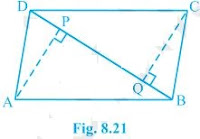10. ABCD is a parallelogram and AP and CQ are perpendiculars from vertices A and C on diagonal BD (see Fig. 8.21). Show that
(i) ΔAPB ≅ ΔCQD
(ii) AP = CQ

(i) In ΔAPB and ΔCQD,
∠ABP = ∠CDQ (Alternate interior angles)
∠APB = ∠CQD (equal to right angles as AP and CQ are perpendiculars)
AB = CD (ABCD is a parallelogram)
Thus, ΔAPB ≅ ΔCQD by AAS congruence condition.

(ii) AP = CQ by CPCT as ΔAPB ≅ ΔCQD.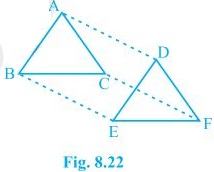11. In ΔABC and ΔDEF, AB = DE, AB || DE, BC = EF and BC || EF. Vertices A, B and C are joined to vertices D, E and F respectively (see Fig. 8.22).
Show that
(i) quadrilateral ABED is a parallelogram
(ii) quadrilateral BEFC is a parallelogram
(iv) quadrilateral ACFD is a parallelogram
(v) AC = DF
(vi) ΔABC ≅ ΔDEF.

(i) AB = DE and AB || DE (Given)
Thus, quadrilateral ABED is a parallelogram because two opposite sides of a quadrilateral are equal and parallel to each other.
(ii) Again BC = EF and BC || EF.
Thus, quadrilateral BEFC is a parallelogram.
(iii)  Since ABED and BEFC are parallelograms.
⇒ AD = BE and BE = CF (Opposite sides of a parallelogram are equal)
Also, AD || BE and BE || CF (Opposite sides of a parallelogram are parallel)

(iv) AD and CF are opposite sides of quadrilateral ACFD which are equal and parallel to each other. Thus, it is a parallelogram.

(v) AC || DF and AC = DF because ACFD is a parallelogram.
(vi) In ΔABC and ΔDEF,
AB = DE (Given)
BC = EF (Given)
AC = DF (Opposite sides of a parallelogram)
Thus, ΔABC ≅ ΔDEF by SSS congruence condition.

12. ABCD is a trapezium in which AB || CD and
AD = BC (see Fig. 8.23). Show that
(i) ∠A = ∠B
(ii) ∠C = ∠D
(iv) diagonal AC = diagonal BD
[Hint : Extend AB and draw a line through C parallel to DA intersecting AB produced at E.]

Construction: Draw a line through C parallel to DA intersecting AB produced at E.
(i) CE = AD (Opposite sides of a parallelogram)
Therefor, BC = CE
⇒ ∠CBE = ∠CEB
also,
∠A ∠CBE = 180° (Angles on the same side of transversal and ∠CBE = ∠CEB)
∠B + ∠CBE = 180° (Linear pair)
⇒ ∠A = ∠B

(ii) ∠A ∠D = ∠B ∠C = 180° (Angles on the same side of transversal)
⇒ ∠A + ∠D = ∠A + ∠C (∠A = ∠B)
⇒ ∠D = ∠C

AB = AB (Common)
∠DBA = ∠CBA
Thus, ΔABC ≅ ΔBAD by SAS congruence condition.

(iv) Diagonal AC = diagonal BD by CPCT as ΔABC ≅ ΔBA.

Page No: 150

Exercise 8.2

1. ABCD is a quadrilateral in which P, Q, R and S are mid-points of the sides AB, BC, CD and DA (see Fig 8.29). AC is a diagonal. Show that :
(i) SR || AC and SR = 1/2 AC
(ii) PQ = SR
(iii) PQRS is a parallelogram.(i) In ΔDAC,
R is the mid point of DC and S is the mid point of DA.
Thus by mid point theorem, SR || AC and SR = 1/2 AC

(ii) In ΔBAC,
P is the mid point of AB and Q is the mid point of BC.
Thus by mid point theorem, PQ || AC and PQ = 1/2 AC
also, SR = 1/2 AC
Thus, PQ = SR

(iii) SR || AC - from (i)
and, PQ || AC - from (ii)
⇒ SR || PQ - from (i) and (ii)
also, PQ = SR
Thus, PQRS is a parallelogram.

2. ABCD is a rhombus and P, Q, R and S are the mid-points of the sides AB, BC, CD and DA respectively. Show that the quadrilateral PQRS is a rectangle.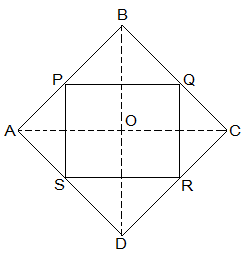Given,
ABCD is a rhombus and P, Q, R and S are the mid-points of the sides AB, BC, CD and DA respectively.
To Prove,
PQRS is a rectangle.
Construction,
AC and BD are joined.
Proof,
In ΔDRS and ΔBPQ,
DS = BQ (Halves of the opposite sides of the rhombus)
∠SDR = ∠QBP (Opposite angles of the rhombus)
DR = BP (Halves of the opposite sides of the rhombus)
Thus, ΔDRS ≅ ΔBPQ by SAS congruence condition.
RS = PQ by CPCT --- (i)
In ΔQCR and ΔSAP,
RC = PA (Halves of the opposite sides of the rhombus)
∠RCQ = ∠PAS (Opposite angles of the rhombus)
CQ = AS (Halves of the opposite sides of the rhombus)
Thus, ΔQCR ≅ ΔSAP by SAS congruence condition.
RQ = SP by CPCT --- (ii)
Now,
In ΔCDB,
R and Q are the mid points of CD and BC respectively.
⇒ QR || BD
also,
P and S are the mid points of AD and AB respectively.
⇒ PS || BD
⇒ QR || PS
Thus, PQRS is a parallelogram.
also, ∠PQR = 90°
Now,
In PQRS,
RS = PQ and RQ = SP from (i) and (ii)
∠Q = 90°
Thus, PQRS is a rectangle.

3. ABCD is a rectangle and P, Q, R and S are mid-points of the sides AB, BC, CD and DA respectively. Show that the quadrilateral PQRS is a rhombus.Given,
ABCD is a rectangle and P, Q, R and S are mid-points of the sides AB, BC, CD and DA respectively.
Construction,
AC and BD are joined.
To Prove,
PQRS is a rhombus.
Proof,
In ΔABC
P and Q are the mid-points of AB and BC respectively
Thus, PQ || AC and PQ = 1/2 AC (Mid point theorem) --- (i)
SR || AC and SR = 1/2 AC (Mid point theorem) --- (ii)
So, PQ || SR and PQ = SR
As in quadrilateral PQRS one pair of opposite sides is equal and parallel to each other, so, it is a parallelogram.
PS || QR and PS = QR (Opposite sides of parallelogram) --- (iii)
Now,
In ΔBCD,
Q and R are mid points of side BC and CD respectively.
Thus, QR || BD and QR = 1/2 BD (Mid point theorem) --- (iv)
AC = BD (Diagonals of a rectangle are equal) --- (v)
From equations (i), (ii), (iii), (iv) and (v),
PQ = QR = SR = PS
So, PQRS is a rhombus.

4. ABCD is a trapezium in which AB || DC, BD is a diagonal and E is the mid-point of AD. A line is drawn through E parallel to AB intersecting BC at F (see Fig. 8.30). Show that F is the mid-point of BC.Given,
ABCD is a trapezium in which AB || DC, BD is a diagonal and E is the mid-point of AD.
To prove,
F is the mid-point of BC.
Proof,
BD intersected EF at G.
E is the mid point of AD and also EG || AB.
Thus, G is the mid point of BD (Converse of mid point theorem)
Now,
In ΔBDC,
G is the mid point of BD and also GF || AB || DC.
Thus, F is the mid point of BC (Converse of mid point theorem)

Page No: 151

5. In a parallelogram ABCD, E and F are the mid-points of sides AB and CD respectively (see Fig. 8.31). Show that the line segments AF and EC trisect the diagonal BD.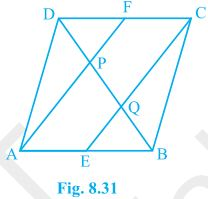Given,
ABCD is a parallelogram. E and F are the mid-points of sides AB and CD respectively.
To show,
AF and EC trisect the diagonal BD.
Proof,
ABCD is a parallelogram
Therefor, AB || CD
also, AE || FC
Now,
AB = CD (Opposite sides of parallelogram ABCD)
⇒ 1/2 AB = 1/2 CD
⇒ AE = FC (E and F are midpoints of side AB and CD)
AECF is a parallelogram (AE and CF are parallel and equal to each other)
AF || EC (Opposite sides of a parallelogram)
Now,
In ΔDQC,
F is mid point of side DC and FP || CQ (as AF || EC).
P is the mid-point of DQ (Converse of mid-point theorem)
⇒ DP = PQ --- (i)
Similarly,
In APB,
E is mid point of side AB and EQ || AP (as AF || EC).
Q is the mid-point of PB (Converse of mid-point theorem)
⇒ PQ = QB --- (ii)
From equations (i) and (i),
DP = PQ = BQ
Hence, the line segments AF and EC trisect the diagonal BD.

6. Show that the line segments joining the mid-points of the opposite sides of a quadrilateral bisect each other.Let ABCD be a quadrilateral and P, Q, R and S are the mid points of AB, BC, CD and DA respectively.
Now,
In ΔACD,
R and S are the mid points of CD and DA respectively.
Thus, SR || AC.
Similarly we can show that,
PQ || AC
PS || BD
QR || BD
Thus, PQRS is parallelogram.
PR and QS are the diagonals of the parallelogram PQRS. So, they will bisect each other.

7. ABC is a triangle right angled at C. A line through the mid-point M of hypotenuse AB and parallel to BC intersects AC at D. Show that
(i) D is the mid-point of AC

(ii) MD ⊥ AC
(iii) CM = MA = 1/2 AB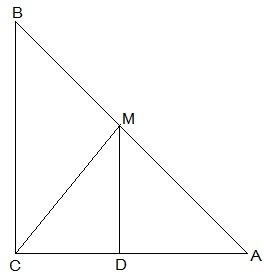(i) In ΔACB,
M is the mid point of AB and MD || BC
Thus, D is the mid point of AC (Converse of mid point theorem)

(ii) ∠ACB = ∠ADM (Corresponding angles)
also, ∠ACB = 90°
Thus, ∠ADM = 90° and MD ⊥ AC

(iii)  In ΔAMD and ΔCMD,
AD = CD (D is the midpoint of side AC)